# Chapter 16 Basic Data Structures Chapter Goals To

• Slides: 90Chapter 16 – Basic Data StructuresChapter Goals To understand the implementation of linked lists and array lists To analyze the efficiency of fundamental operations of lists and arrays To implement the stack and queue data types To implement a hash table and understand the efficiency of its operations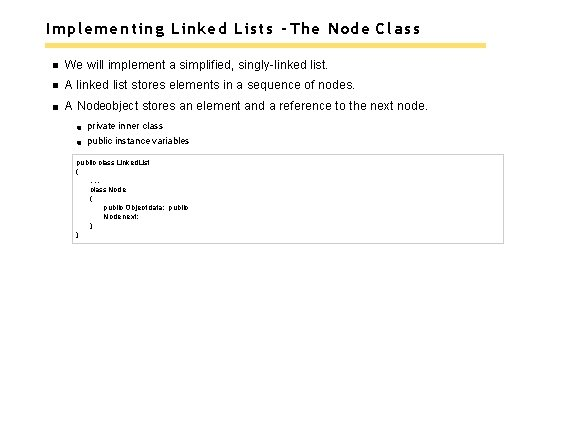Implementing Linked Lists - The Node Class We will implement a simplified, singly-linked list. A linked list stores elements in a sequence of nodes. A Nodeobject stores an element and a reference to the next node. private inner class public instance variables public class Linked. List {. . . class Node { public Object data; public Node next; } }Implementing Linked Lists - The Node Class A linked list object holds a reference to the first node: Each node holds a reference to the next node. public class Linked. List { private Node first; public Linked. List() { first = null; } public Object get. First() { if (first == null) { throw new No. Such. Element. Exception(); } return first. data; } }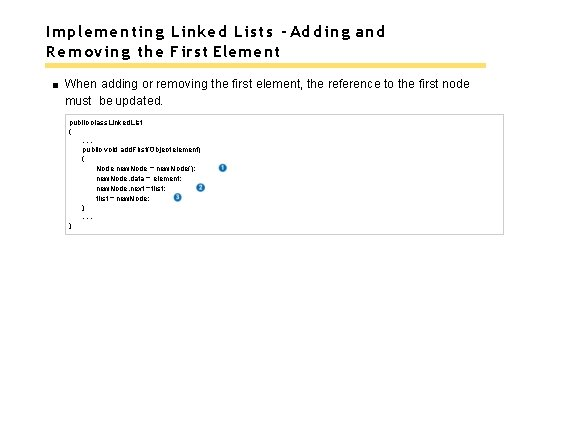Implementing Linked Lists - Adding and Removing the First Element When adding or removing the first element, the reference to the first node must be updated. public class Linked. List {. . . public void add. First(Object element) { Node new. Node = new Node(); new. Node. data = element; new. Node. next = first; first = new. Node; }. . . }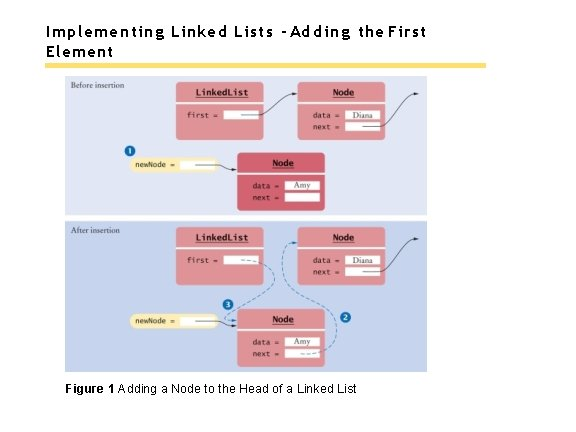Implementing Linked Lists - Removing the First Element The data of the first node are saved and later returned as the method result. The successor of the first node becomes the first node of the shorter list. The old node is eventually recycled by the garbage collector. public class Linked. List {. . . public Object remove. First() { if (first == null) { throw new No. Such. Element. Exception(); } Object element = first. data; first = first. next; return element; }. . . }Implementing Linked Lists - Removing the First Element Figure 2 Removing the First Node from a Linked. ListThe Iterator Class Our simplified List. Iteratorinterface has methods: next, has. Next, remove, add, and set. Our Linked. Listclass declares a private inner class Linked. List. Iteratorimplements our simplified List. Iteratorinterface. As an inner class Linked. List. Iteratorhas access to The instance variable first The private Nodeclass. A list iterator object has: A reference to the currently visited node, position A reference to the last node before that, previous A is. After. Nextflag to track when the nextmethod has been called.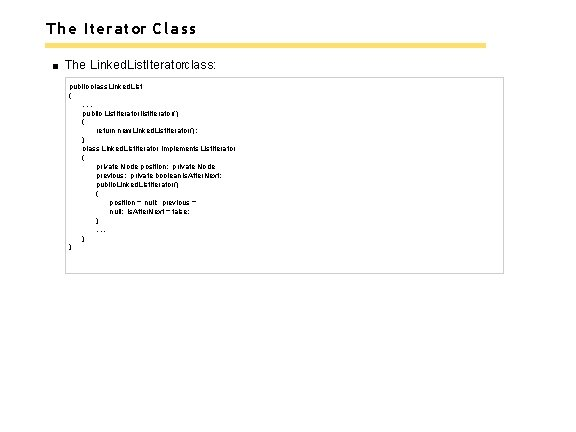The Iterator Class The Linked. List. Iteratorclass: public class Linked. List {. . . public List. Iterator list. Iterator() { return new Linked. List. Iterator(); } class Linked. List. Iterator implements List. Iterator { private Node position; private Node previous; private boolean is. After. Next; public Linked. List. Iterator() { position = null; previous = null; is. After. Next = false; }. . . } }Advancing an Iterator To advance an iterator: Update the position Remember the old position for the removemethod. The nextmethod: class Linked. List. Iterator implements. List. Iterator {. . . public Object next() { if (!has. Next()) { throw new No. Such. Element. Exception(); } previous = position; // Remember for remove is. After. Next = true; if (position == null) { position = first; } else { position = position. next; } return position. data; }. . . }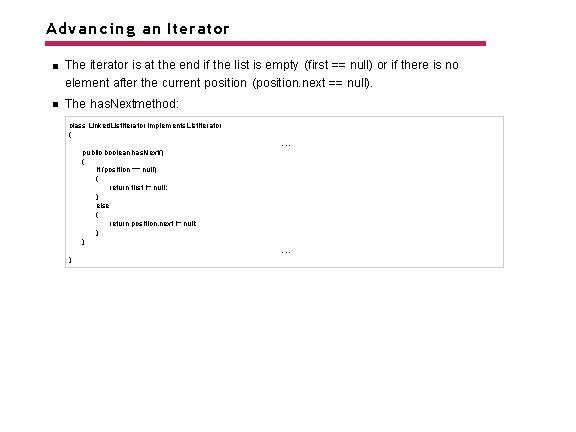Advancing an Iterator The iterator is at the end if the list is empty (first == null) or if there is no element after the current position (position. next == null). The has. Nextmethod: class Linked. List. Iterator implements List. Iterator {. . . public boolean has. Next() { if (position == null) { return first != null; } else { return position. next != null; } }. . . }Removing an Element If this is the first element: Call remove. First Otherwise, update the nextreference of the previous node Update is. After. Nextto disallow another call to remove. The removemethod: class Linked. List. Iterator implements. List. Iterator {. . . public void remove() { if (!is. After. Next) { throw new Illegal. State. Exception(); } if (position == first) { remove. First(); } else { previous. next = position. next; } position = previous; is. After. Next = false; }. . . }Removing an Element Figure 3 Removing a Node from the Middle of a Linked List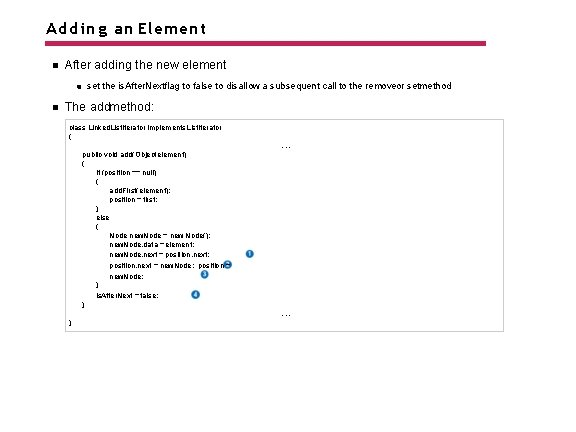Adding an Element After adding the new element set the is. After. Nextflag to false to disallow a subsequent call to the removeor setmethod The addmethod: class Linked. List. Iterator implements List. Iterator {. . . public void add(Object element) { if (position == null) { add. First(element); position = first; } else { Node new. Node = new Node(); new. Node. data = element; new. Node. next = position. next; position. next = new. Node; position = new. Node; } is. After. Next = false; }. . . }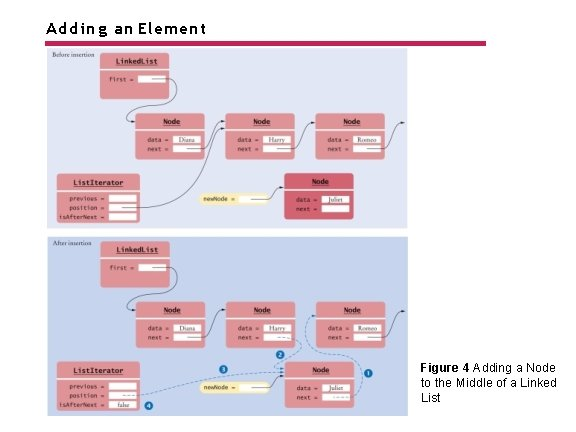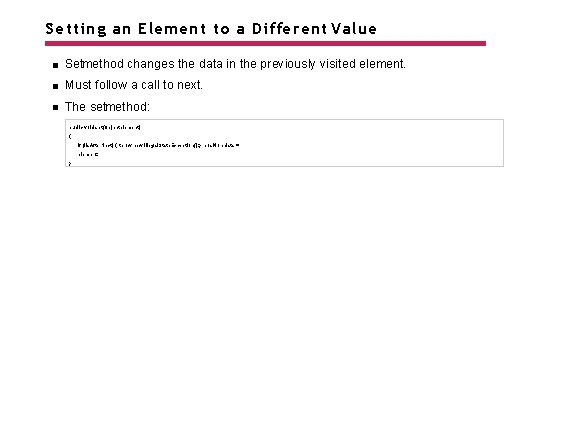Setting an Element to a Different Value Setmethod changes the data in the previously visited element. Must follow a call to next. The setmethod: public void set(Object element) { if (!is. After. Next) { throw new Illegal. State. Exception(); } position. data = element; }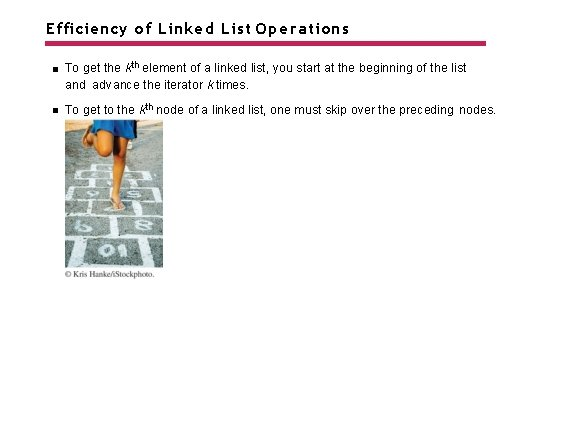Efficiency of Linked List Operations To get the kth element of a linked list, you start at the beginning of the list and advance the iterator k times. To get to the kth node of a linked list, one must skip over the preceding nodes.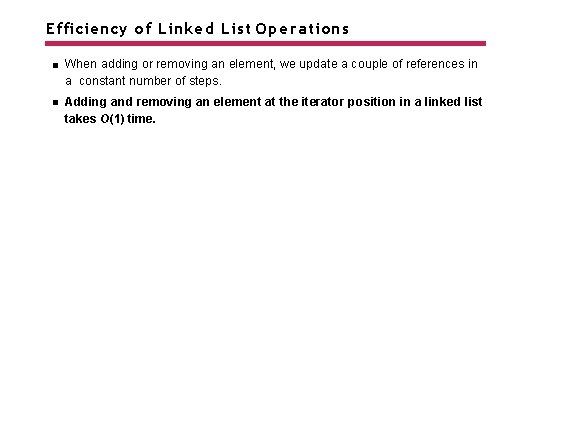Efficiency of Linked List Operations When adding or removing an element, we update a couple of references in a constant number of steps. Adding and removing an element at the iterator position in a linked list takes O(1) time.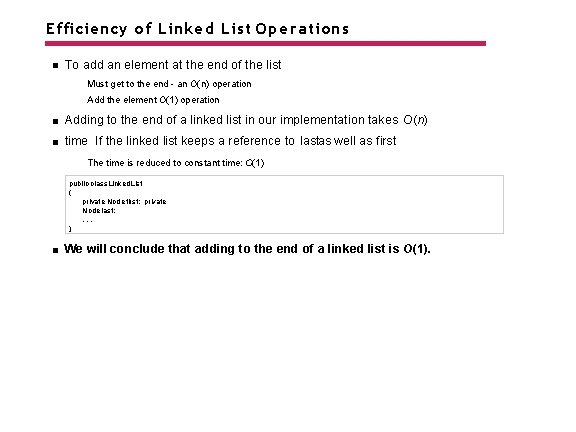Efficiency of Linked List Operations To add an element at the end of the list Must get to the end - an O(n) operation Add the element O(1) operation Adding to the end of a linked list in our implementation takes O(n) time If the linked list keeps a reference to lastas well as first The time is reduced to constant time: O(1) public class Linked. List { private Node first; private Node last; . . . } We will conclude that adding to the end of a linked list is O(1).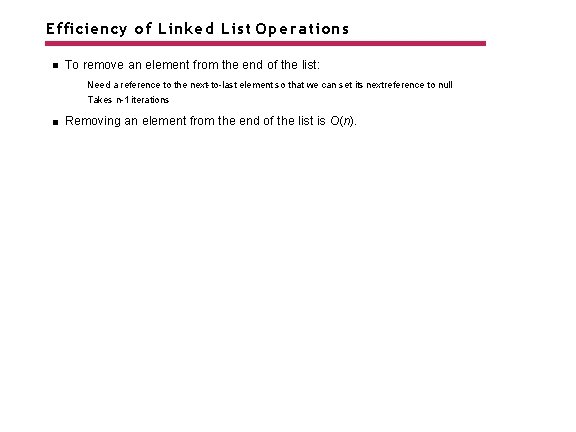Efficiency of Linked List Operations To remove an element from the end of the list: Need a reference to the next-to-last element so that we can set its next reference to null Takes n-1 iterations Removing an element from the end of the list is O(n).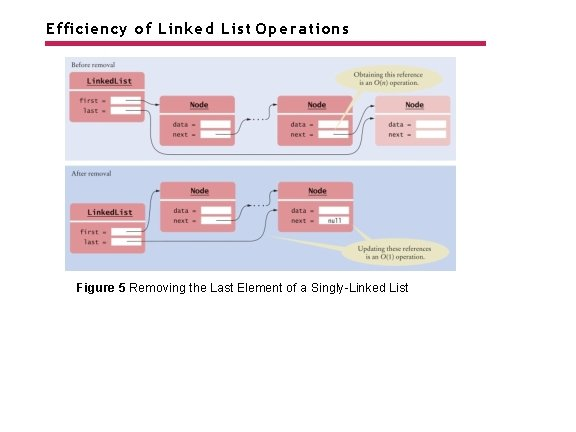Efficiency of Linked List Operations Figure 5 Removing the Last Element of a Singly-Linked List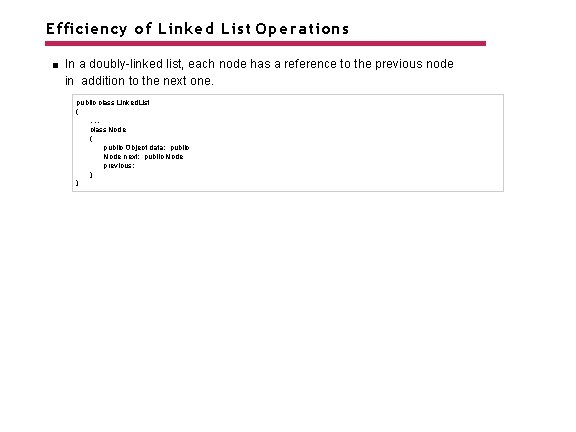Efficiency of Linked List Operations In a doubly-linked list, each node has a reference to the previous node in addition to the next one. public class Linked. List {. . . class Node { public Object data; public Node next; public Node previous; } }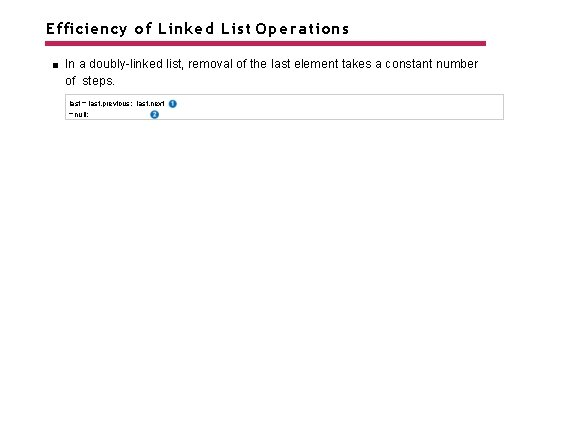Efficiency of Linked List Operations In a doubly-linked list, removal of the last element takes a constant number of steps. last = last. previous; last. next = null;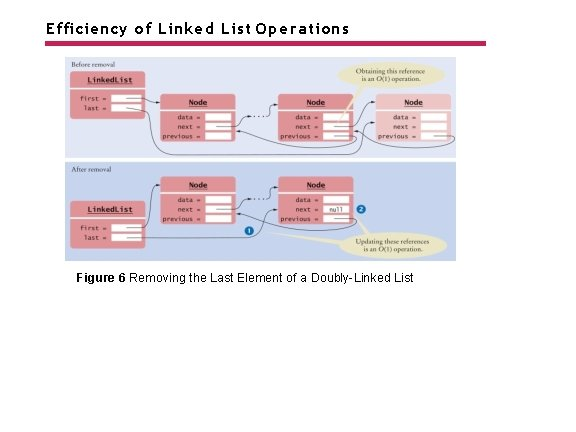Efficiency of Linked List Operations Figure 6 Removing the Last Element of a Doubly-Linked List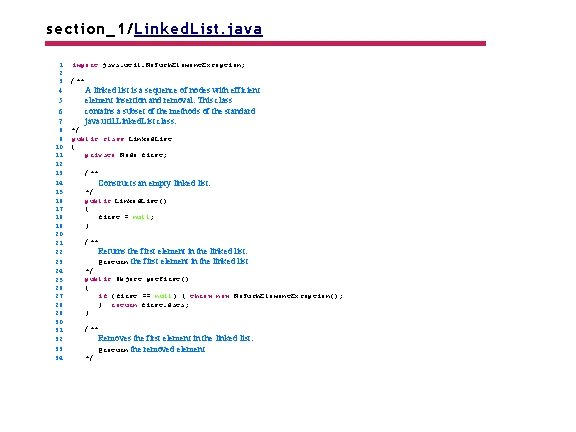section_1/Linked. List. java 1 2 3 4 5 6 7 8 9 10 11 12 13 14 15 16 17 18 19 20 21 22 23 24 25 26 27 28 29 30 31 32 33 34 import java. util. No. Such. Element. Exception; /** A linked list is a sequence of nodes with efficient element insertion and removal. This class contains a subset of the methods of the standard java. util. Linked. List class. */ public class Linked. List { private Node first; /** Constructs an empty linked list. */ public Linked. List() { first = null; } /** Returns the first element in the linked list. @return the first element in the linked list */ public Object get. First() { if (first == null) { throw new No. Such. Element. Exception(); } return first. data; } /** */ Removes the first element in the linked list. @return the removed elementsection_1/List. Iterator. java 1 2 3 4 5 6 7 8 9 /** A list iterator allows access of a position in a linked list. This interface contains a subset of the methods of the standard java. util. List. Iterator interface. The methods for backward traversal are not included. */ public interface List. Iterator { /** Moves the iterator past the next element. @return the traversed element 10 11 12 13 14 15 16 17 18 19 20 21 22 23 24 25 26 27 28 29 30 31 32 33 */ Object next(); /** Tests if there is an element after the iterator position. @return true if there is an element after the iterator position */ boolean has. Next(); /** Adds an element before the iterator position and moves the iterator past the inserted element. @param element the element to add */ void add(Object element); /** Removes the last traversed element. This method may only be called after a call to the next() method. */ void remove();Self Check 16. 1 Trace through the add. Firstmethod when adding an element to an empty list. Answer: When the list is empty, firstis null. A new Nodeis allocated. Its data instance variable is set to the element that is being added. It’s next instance variable is set to nullbecause firstis null. The firstinstance variable is set to the new node. The result is a linked list of length 1.Self Check 16. 2 Conceptually, an iterator is located between two elements (see Figure 9 in Chapter 15). Does the positioninstance variable refer to the element to the left or the element to the right? Answer: It refers to the element to the left. You can see that by tracing out the first call to next. It leaves positionto refer to the first node.Self Check 16. 3 Why does the addmethod have two separate cases? Answer: If positionis null, we must be at the head of the list, and inserting an element requires updating the first reference. If we are in the middle of the list, the firstreference should not be changed.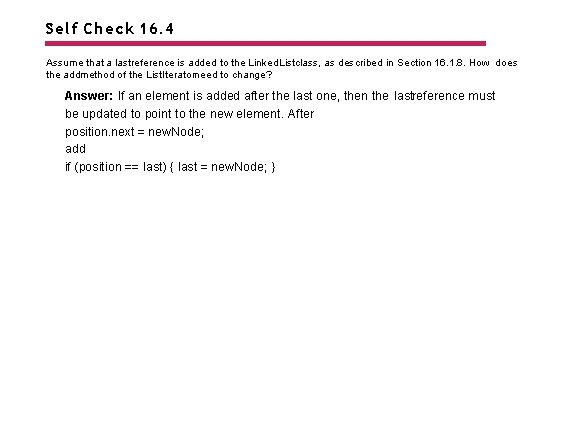Self Check 16. 4 Assume that a lastreference is added to the Linked. Listclass, as described in Section 16. 1. 8. How does the addmethod of the List. Iteratorneed to change? Answer: If an element is added after the last one, then the lastreference must be updated to point to the new element. After position. next = new. Node; add if (position == last) { last = new. Node; }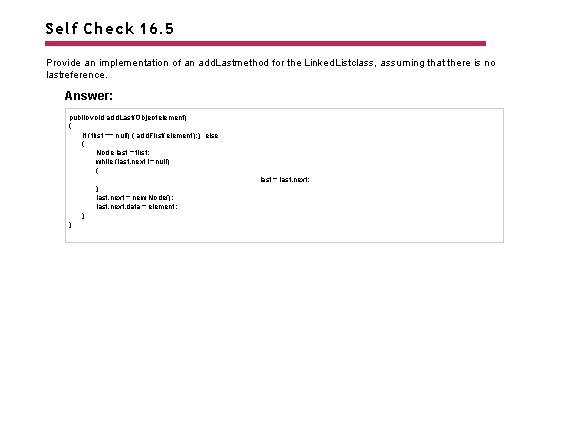Self Check 16. 5 Provide an implementation of an add. Lastmethod for the Linked. Listclass, assuming that there is no lastreference. Answer: public void add. Last(Object element) { if (first == null) { add. First(element); } else { Node last = first; while (last. next != null) { last = last. next; } last. next = new Node(); last. next. data = element; } }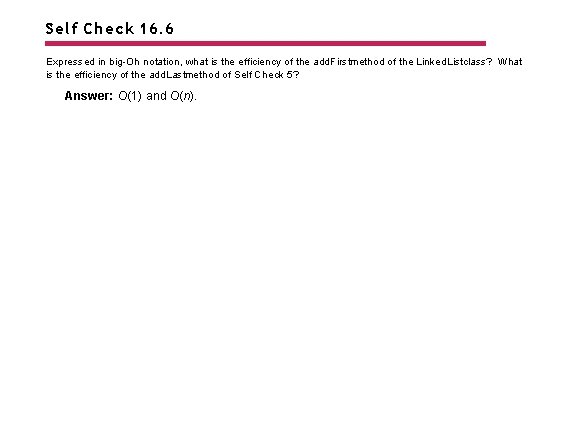Self Check 16. 6 Expressed in big-Oh notation, what is the efficiency of the add. Firstmethod of the Linked. Listclass? What is the efficiency of the add. Lastmethod of Self Check 5? Answer: O(1) and O(n).Self Check 16. 7 How much slower is the binary search algorithm for a linked list compared to the linear search algorithm? Answer: To locate the middle element takes n / 2 steps. To locate the middle of the subinterval to the left or right takes another n / 4 steps. The next lookup takes n / 8 steps. Thus, we expect almost n steps to locate an element. At this point, you are better off just making a linear search that, on average, takes n / 2 steps.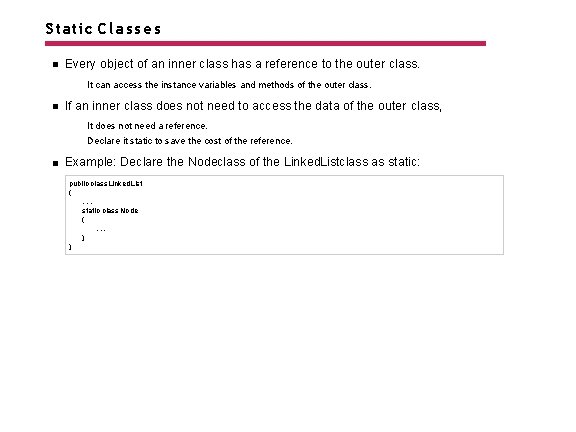Static Classes Every object of an inner class has a reference to the outer class. It can access the instance variables and methods of the outer class. If an inner class does not need to access the data of the outer class, It does not need a reference. Declare it static to save the cost of the reference. Example: Declare the Nodeclass of the Linked. Listclass as static: public class Linked. List {. . . static class Node {. . . } }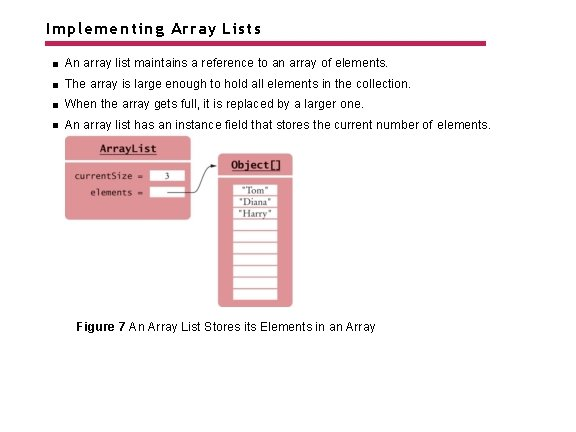Implementing Array Lists An array list maintains a reference to an array of elements. The array is large enough to hold all elements in the collection. When the array gets full, it is replaced by a larger one. An array list has an instance field that stores the current number of elements. Figure 7 An Array List Stores its Elements in an Array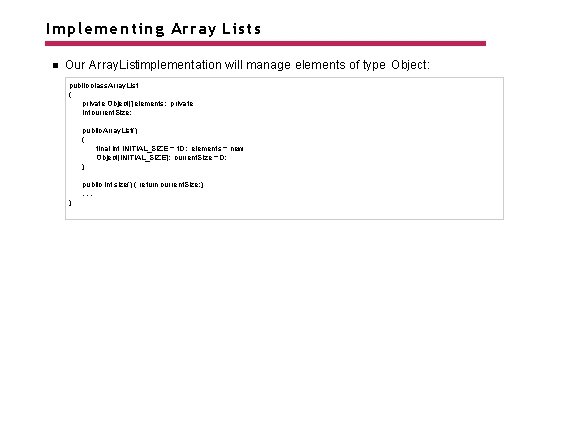Implementing Array Lists Our Array. Listimplementation will manage elements of type Object: public class Array. List { private Object[] elements; private int current. Size; public Array. List() { final int INITIAL_SIZE = 10; elements = new Object[INITIAL_SIZE]; current. Size = 0; } public int size() { return current. Size; }. . . }Implementing Array Lists - Getting and Setting Elements Providing getand setmethods: Check for valid positions Access the internal array at the given position Helper method to check bounds: private void check. Bounds(int n) { if (n < 0 || n >= current. Size) { throw new Index. Out. Of. Bounds. Exception(); } }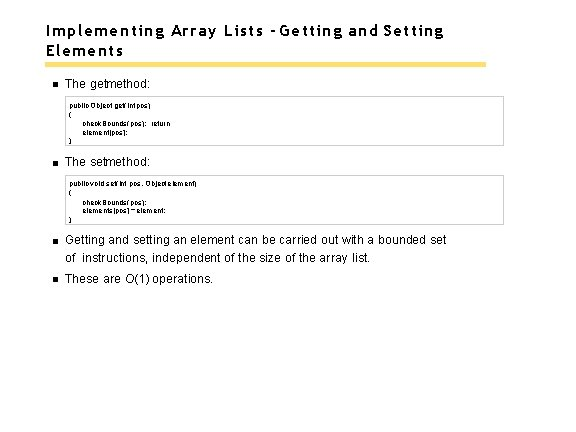Implementing Array Lists - Getting and Setting Elements The getmethod: public Object get(int pos) { check. Bounds(pos); return element[pos]; } The setmethod: public void set(int pos, Object element) { check. Bounds(pos); elements[pos] = element; } Getting and setting an element can be carried out with a bounded set of instructions, independent of the size of the array list. These are O(1) operations.Removing or Adding Elements To remove an element at position k, move the elements with higher index values. The removemethod: public Object remove(int pos) { check. Bounds(pos); Object removed = elements[pos]; for (int i = pos + 1; i < current. Size; i++) { elements[i - 1] = elements[i]; } current. Size--; return removed; } On average, n / 2 elements need to move. Inserting a element also requires moving, on average, n /2 elements. Inserting or removing an array list element is an O(n) operation.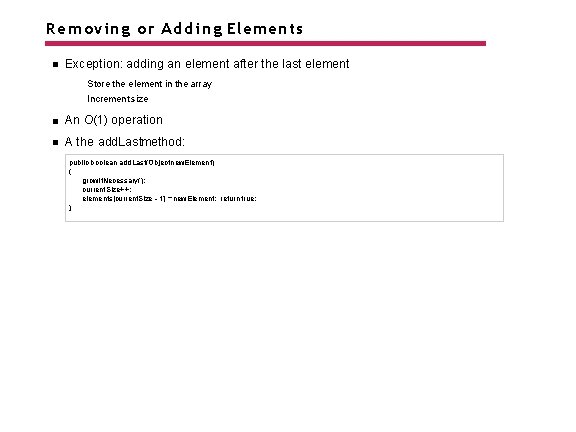Removing or Adding Elements Exception: adding an element after the last element Store the element in the array Increment size An O(1) operation A the add. Lastmethod: public boolean add. Last(Object new. Element) { grow. If. Necessary(); current. Size++; elements[current. Size - 1] = new. Element; return true; }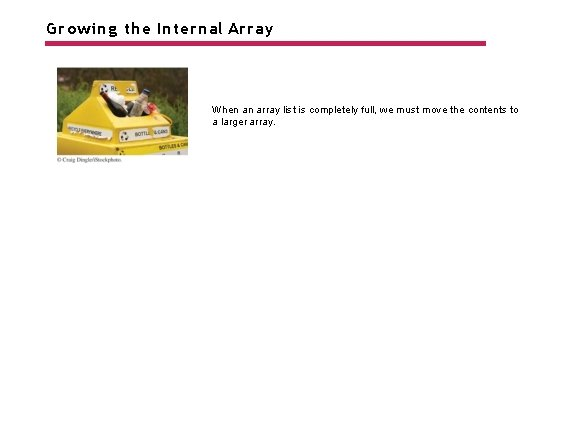Growing the Internal Array When an array list is completely full, we must move the contents to a larger array.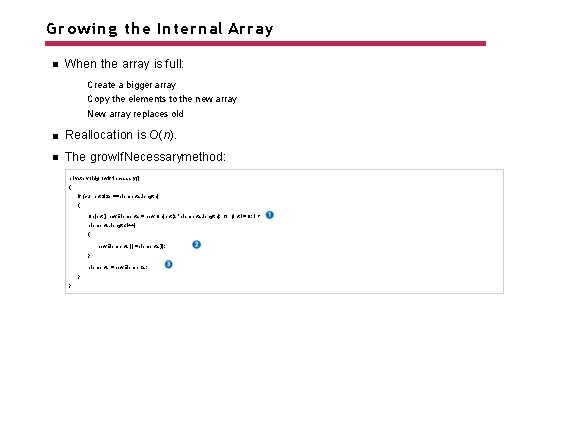Growing the Internal Array When the array is full: Create a bigger array Copy the elements to the new array New array replaces old Reallocation is O(n). The grow. If. Necessarymethod: private void grow. If. Necessary() { if (current. Size == elements. length) { Object[] new. Elements = new Object[2 * elements. length]; for (int i = 0; i < elements. length; i++) { new. Elements[i] = elements[i]; } elements = new. Elements; } }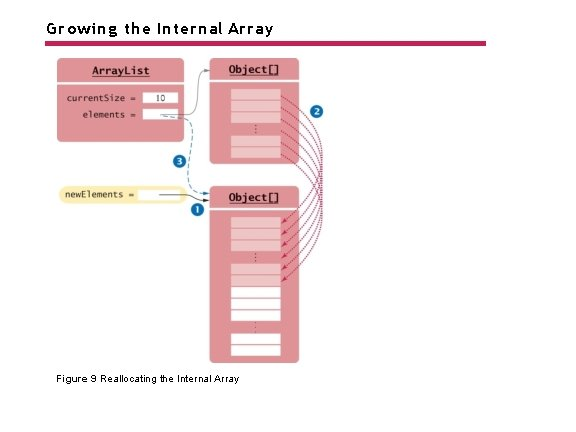Growing the Internal Array Figure 9 Reallocating the Internal Array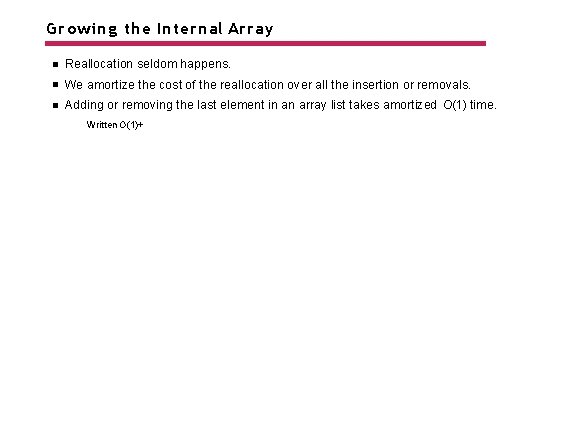Growing the Internal Array Reallocation seldom happens. We amortize the cost of the reallocation over all the insertion or removals. Adding or removing the last element in an array list takes amortized O(1) time. Written O(1)+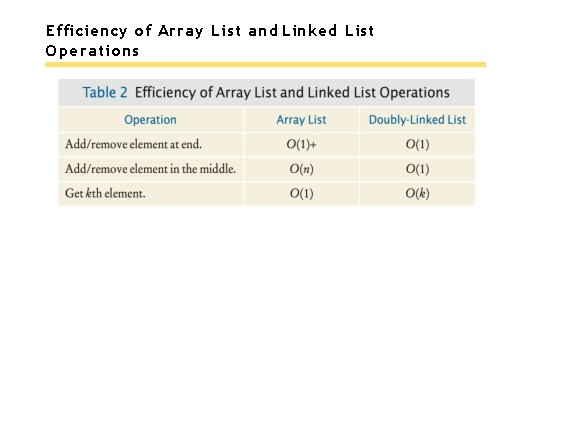Efficiency of Array List and Linked List Operations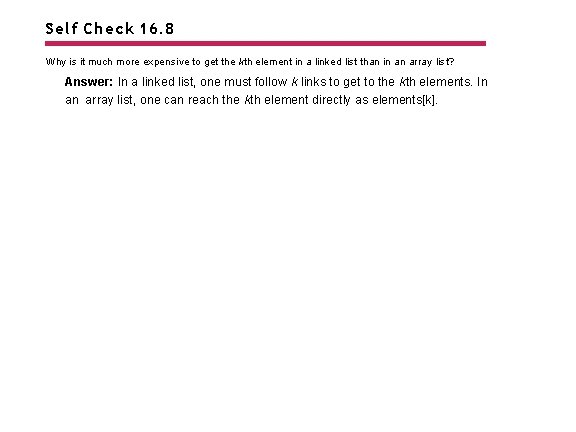Self Check 16. 8 Why is it much more expensive to get the kth element in a linked list than in an array list? Answer: In a linked list, one must follow k links to get to the kth elements. In an array list, one can reach the kth element directly as elements[k].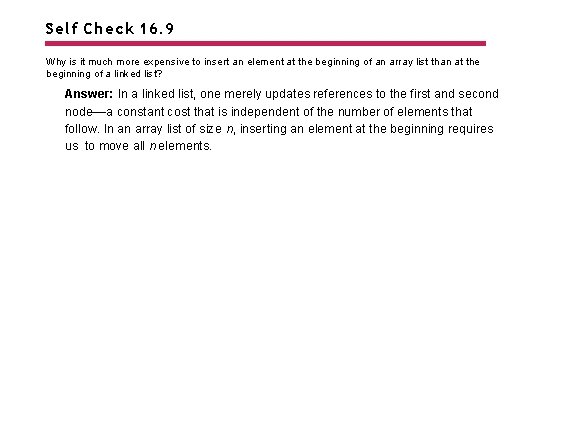Self Check 16. 9 Why is it much more expensive to insert an element at the beginning of an array list than at the beginning of a linked list? Answer: In a linked list, one merely updates references to the first and second node––a constant cost that is independent of the number of elements that follow. In an array list of size n, inserting an element at the beginning requires us to move all n elements.Self Check 16. 10 What is the efficiency of adding an element exactly in the middle of a linked list? An array list? Answer: It is O(n) in both cases. In the case of the linked list, it costs O(n) steps to move an iterator to the middle.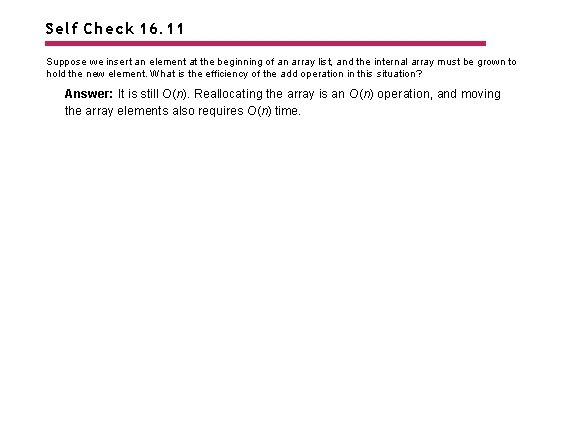Self Check 16. 11 Suppose we insert an element at the beginning of an array list, and the internal array must be grown to hold the new element. What is the efficiency of the add operation in this situation? Answer: It is still O(n). Reallocating the array is an O(n) operation, and moving the array elements also requires O(n) time.Self Check 16. 12 Using big-Oh notation, what is the cost of adding an element to an array list as the second-to-last element? Answer: O(1)+. The cost of moving one element is O(1), but every so often one has to pay for a reallocation.Implementing Stacks and Queues Stacks and queues are abstract data types. We specify how operations must behave. We do not specify the implementation. Many different implementations are possible.Stacks as Linked Lists A stack can be implemented as a sequence of nodes. New elements are “pushed” to one end of the sequence, and they are “popped” from the same end. Push and pop from the least expensive end - the front. The pushand popoperations are identical to the add. Firstand remove. Firstoperations of the linked list.Stacks as Linked Lists Figure 10 Push and Pop for a Stack Implemented as a Linked Lists ection_3_1/ Linked. Lis t. Stack. java 1 2 3 4 5 6 7 8 9 10 11 12 13 14 15 16 17 18 19 20 21 22 23 24 25 26 27 28 29 30 31 32 33 34 35 import java. util. No. Such. Element. Exception; /** An implementation of a stack as a sequence of nodes. */ public class Linked. List. Stack { private Node first; /** Constructs an empty stack. */ public Linked. List. Stack() { first = null; } /** Adds an element to the top of the stack. @param element the element to add */ public void push(Object element) { Node new. Node = new Node(); new. Node. data = element; new. Node. next = first; first = new. Node; } /** Removes the element from the top of the stack. @return the removed element */ public Object pop() {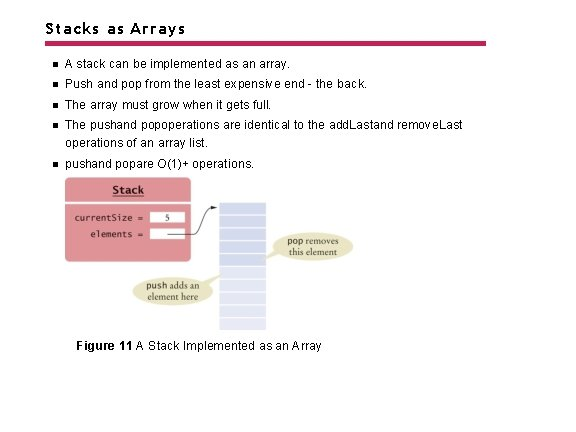Stacks as Arrays A stack can be implemented as an array. Push and pop from the least expensive end - the back. The array must grow when it gets full. The pushand popoperations are identical to the add. Lastand remove. Last operations of an array list. pushand popare O(1)+ operations. Figure 11 A Stack Implemented as an Array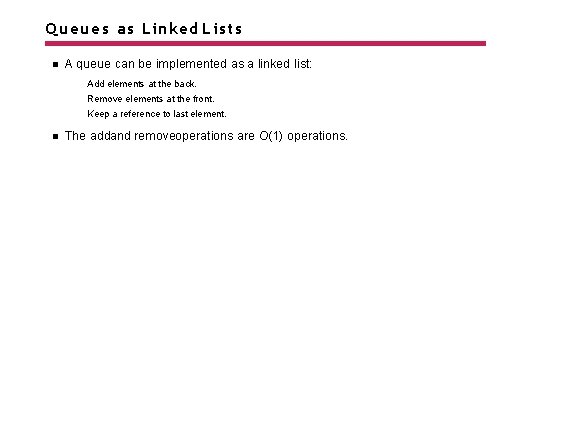Queues as Linked Lists A queue can be implemented as a linked list: Add elements at the back. Remove elements at the front. Keep a reference to last element. The addand removeoperations are O(1) operations.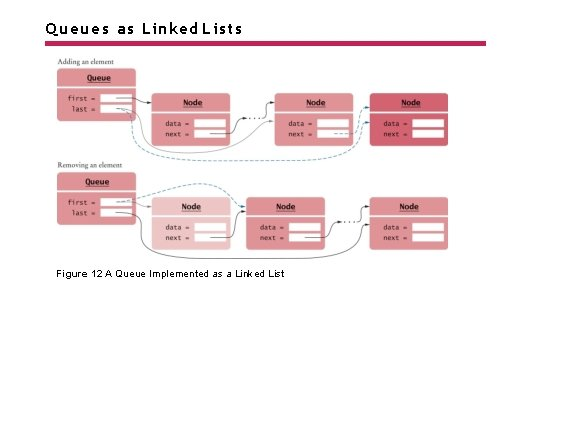Queues as Linked Lists Figure 12 A Queue Implemented as a Linked List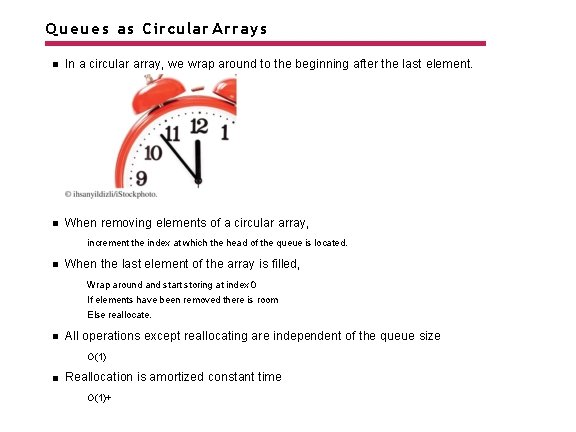Queues as Circular Arrays In a circular array, we wrap around to the beginning after the last element. When removing elements of a circular array, increment the index at which the head of the queue is located. When the last element of the array is filled, Wrap around and start storing at index 0 If elements have been removed there is room Else reallocate. All operations except reallocating are independent of the queue size O(1) Reallocation is amortized constant time O(1)+Queues as Circular Arrays Figure 13 Queue Elements in a Circular ArrayQueues as Circular Arrays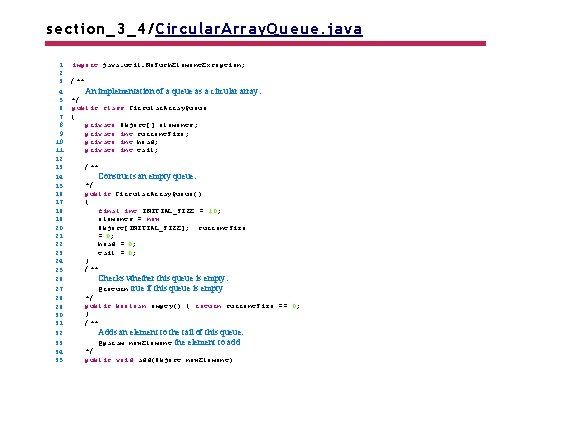section_3_4/Circular. Array. Queue. java 1 2 3 4 5 6 7 8 9 10 11 12 13 14 15 16 17 18 19 20 21 22 23 24 25 26 27 28 29 30 31 32 33 34 35 import java. util. No. Such. Element. Exception; /** An implementation of a queue as a circular array. */ public class Circular. Array. Queue { private Object[] elements; private int current. Size; private int head; private int tail; /** Constructs an empty queue. */ public Circular. Array. Queue() { final int INITIAL_SIZE = 10; elements = new Object[INITIAL_SIZE]; current. Size = 0; head = 0; tail = 0; } /** Checks whether this queue is empty. @return true if this queue is empty */ public boolean empty() { return current. Size == 0; } /** Adds an element to the tail of this queue. @param new. Element the element to add */ public void add(Object new. Element)Self Check 16. 13 Add a method peekto the Stackimplementation in Section 16. 3. 1 that returns the top of the stack without removing it. Answer: public Object peek() { if (first == null) { throw new No. Such. Element. Exception(); } return first. data; }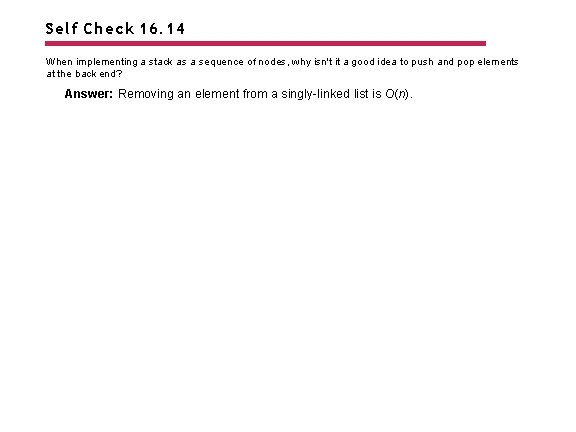Self Check 16. 14 When implementing a stack as a sequence of nodes, why isn't it a good idea to push and pop elements at the back end? Answer: Removing an element from a singly-linked list is O(n).Self Check 16. 15 When implementing a stack as an array, why isn’t it a good idea to push and pop elements at index 0? Answer: Adding and removing an element at index 0 is O(n).Self Check 16. 16 What is wrong with this implementation of the emptymethod for the circular array queue? public boolean empty() { return head == 0 && tail == 0; } Answer: The queue can be empty when the head and tail are at a position other than zero. For example, after the calls q. add(obj) and q. remove(), the queue is empty, but head and tail are 1.Self Check 16. 17 What is wrong with this implementation of the emptymethod for the circular array queue? public boolean empty() { return head == tail; } Answer: Indeed, if the queue is empty, then the head and tail are equal. But that situation also occurs when the array is completely full.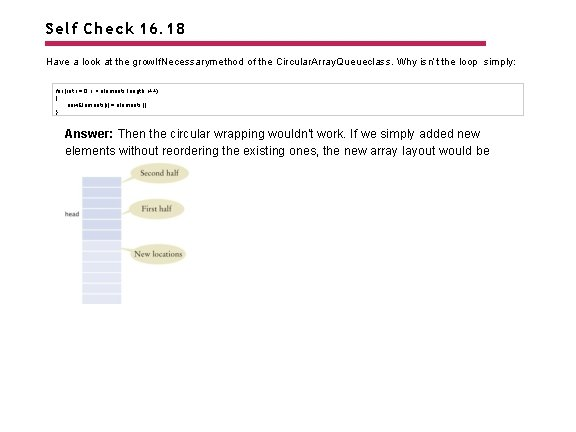Self Check 16. 18 Have a look at the grow. If. Necessarymethod of the Circular. Array. Queueclass. Why isn’t the loop simply: for (int i = 0; i < elements. length; i++) { new. Elements[i] = elements[i]; } Answer: Then the circular wrapping wouldn't work. If we simply added new elements without reordering the existing ones, the new array layout would beImplementing a Hash Table In the Java library sets are implemented as hash sets and tree sets. Hashing: place items into an array at an index determined from the element. Hash code: an integer value that is computed from an object, in such a way that different objects are likely to yield different hash codes. Collision: when two or more distinct objects have the same hash code. A good hash function minimizes collisions. A hash table uses the hash code to determine where to store each element.Implementing a Hash Table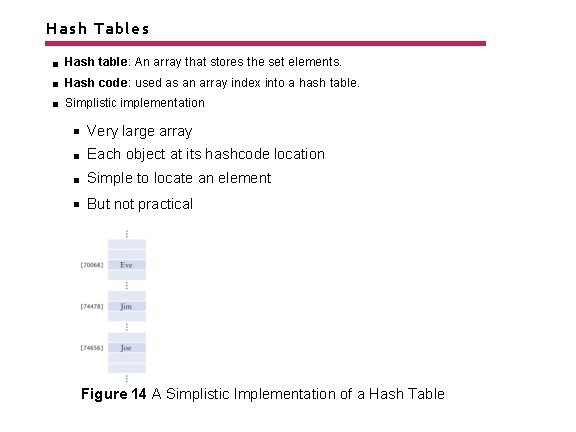Hash Tables Hash table: An array that stores the set elements. Hash code: used as an array index into a hash table. Simplistic implementation Very large array Each object at its hashcode location Simple to locate an element But not practical Figure 14 A Simplistic Implementation of a Hash Table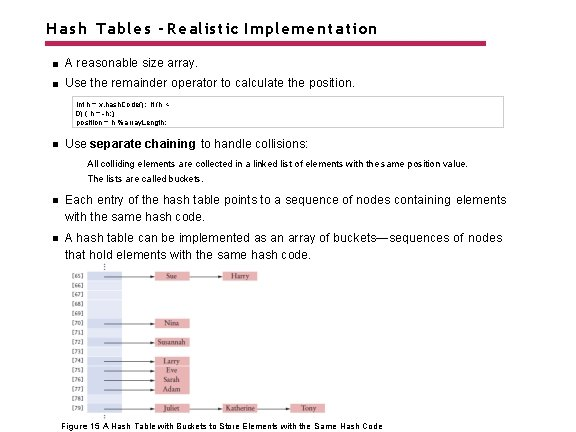Hash Tables - Realistic Implementation A reasonable size array. Use the remainder operator to calculate the position. int h = x. hash. Code(); if (h < 0) { h = -h; } position = h % array. Length; Use separate chaining to handle collisions: All colliding elements are collected in a linked list of elements with the same position value. The lists are called buckets. Each entry of the hash table points to a sequence of nodes containing elements with the same hash code. A hash table can be implemented as an array of buckets—sequences of nodes that hold elements with the same hash code. Figure 15 A Hash Table with Buckets to Store Elements with the Same Hash Code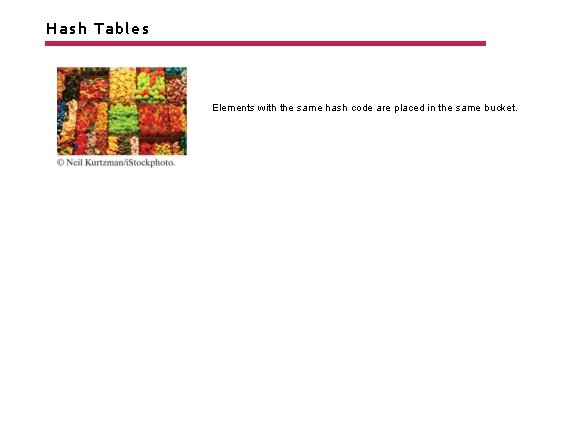Hash Tables Elements with the same hash code are placed in the same bucket.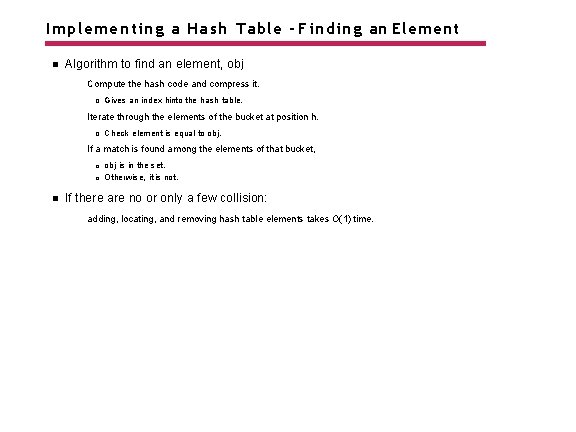Implementing a Hash Table - Finding an Element Algorithm to find an element, obj Compute the hash code and compress it. Gives an index hinto the hash table. Iterate through the elements of the bucket at position h. Check element is equal to obj. If a match is found among the elements of that bucket, obj is in the set. Otherwise, it is not. If there are no or only a few collision: adding, locating, and removing hash table elements takes O(1) time.Adding and Removing Elements Algorithm to add an element: Compute the compressed hash code h. Iterate through the elements of the bucket at position h. For each element of the bucket, check whether it is equal to obj. If a match is found among the elements of that bucket, then exit. Otherwise, add a node containing obj to the beginning of the node sequence. If the load factor exceeds a fixed threshold, reallocate the table. Load factor: a measure of how full the table is. The number of elements in the table divided by the table length. Adding an element to a hash table is O(1)+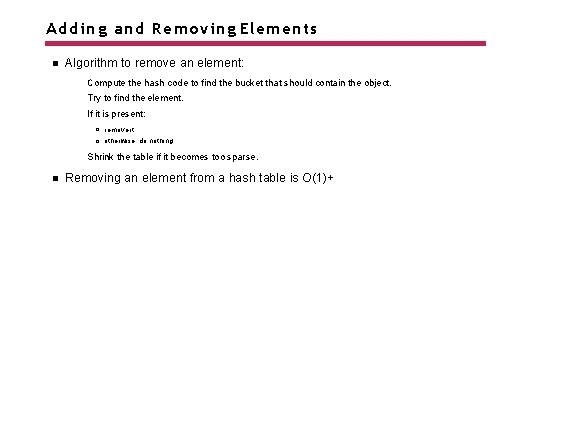Adding and Removing Elements Algorithm to remove an element: Compute the hash code to find the bucket that should contain the object. Try to find the element. If it is present: remove it. otherwise, do nothing. Shrink the table if it becomes too sparse. Removing an element from a hash table is O(1)+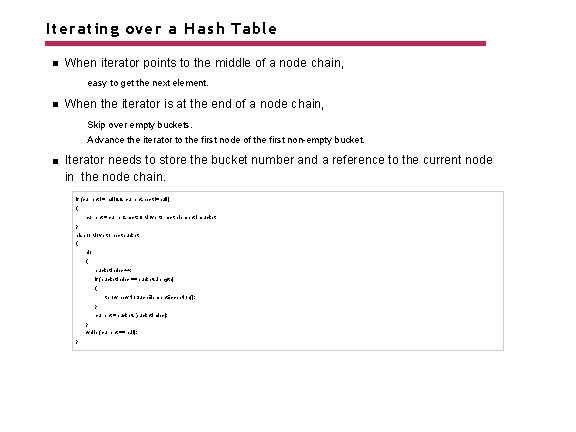Iterating over a Hash Table When iterator points to the middle of a node chain, easy to get the next element. When the iterator is at the end of a node chain, Skip over empty buckets. Advance the iterator to the first node of the first non-empty bucket. Iterator needs to store the bucket number and a reference to the current node in the node chain. if (current != null && current. next !=null) { current = current. next; // Move to next element inbucket } else // Move to next bucket { do { bucket. Index++; if (bucket. Index == buckets. length) { throw new No. Such. Element. Exception(); } current = buckets[bucket. Index]; } while (current == null); }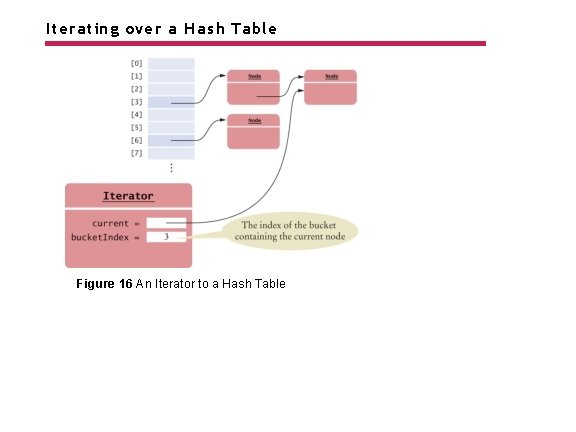Iterating over a Hash Table Figure 16 An Iterator to a Hash Table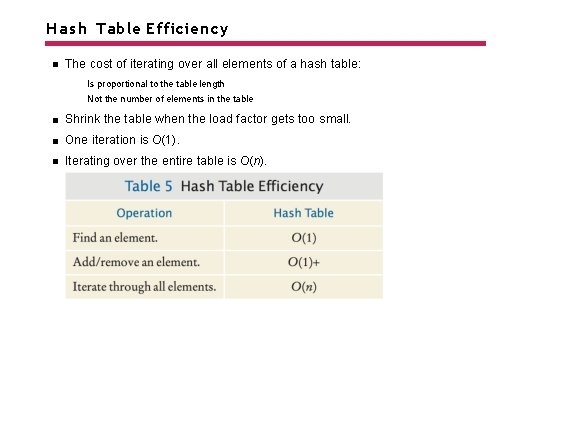Hash Table Efficiency The cost of iterating over all elements of a hash table: Is proportional to the table length Not the number of elements in the table Shrink the table when the load factor gets too small. One iteration is O(1). Iterating over the entire table is O(n).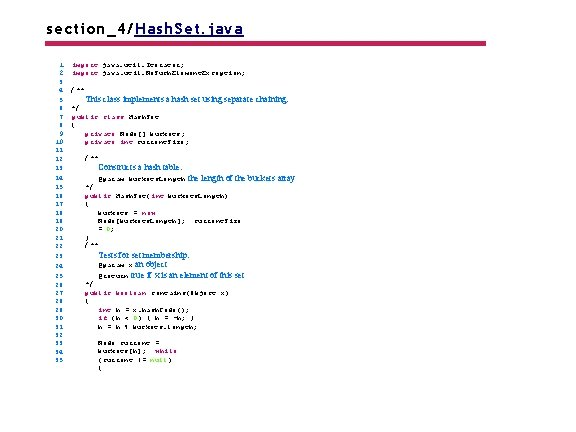section_4/Hash. Set. java 1 2 3 4 5 6 7 8 9 10 11 12 13 14 15 16 17 18 19 20 21 22 23 24 25 26 27 28 29 30 31 32 33 34 35 import java. util. Iterator; import java. util. No. Such. Element. Exception; /** This class implements a hash set using separate chaining. */ public class Hash. Set { private Node[] buckets; private int current. Size; /** Constructs a hash table. @param buckets. Length the length of the */ public Hash. Set(int buckets. Length) { buckets = new Node[buckets. Length]; current. Size = 0; } /** Tests for set membership. @param x an object @return true if x is an element of this set */ public boolean contains(Object x) { int h = x. hash. Code(); if (h < 0) { h = -h; } h = h % buckets. length; Node current = buckets[h]; while (current != null) { buckets array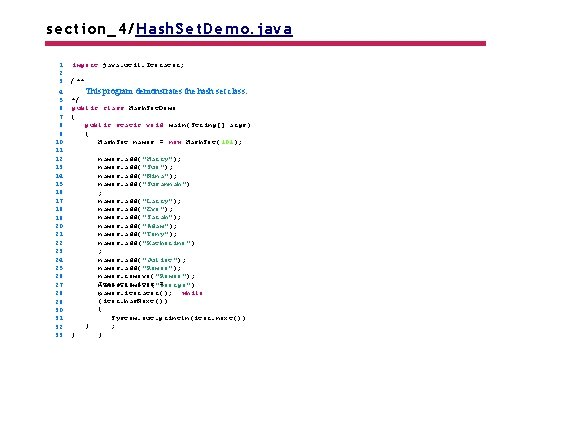section_4/Hash. Set. Demo. java 1 2 3 4 5 6 7 8 9 10 11 12 13 14 15 16 17 18 19 20 21 22 23 24 25 26 27 28 29 30 31 32 33 import java. util. Iterator; /** This program demonstrates the hash set class. */ public class Hash. Set. Demo { public static void main(String[] args) { Hash. Set names = new Hash. Set(101); } } names. add("Harry"); names. add("Sue"); names. add("Nina"); names. add("Susannah") ; names. add("Larry"); names. add("Eve"); names. add("Sarah"); names. add("Adam"); names. add("Tony"); names. add("Katherine") ; names. add("Juliet"); names. add("Romeo"); names. remove( "Romeo"); Iterator iter = names. remove ("George") names. iterator(); while ; (iter. has. Next()) { System. out. println(iter. next()) ; }Program Run: Harry Sue Nina Susannah Larry Eve Sarah Adam Juliet Katherine Tony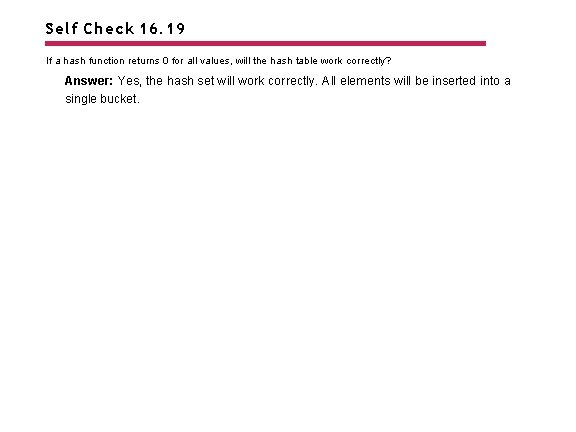Self Check 16. 19 If a hash function returns 0 for all values, will the hash table work correctly? Answer: Yes, the hash set will work correctly. All elements will be inserted into a single bucket.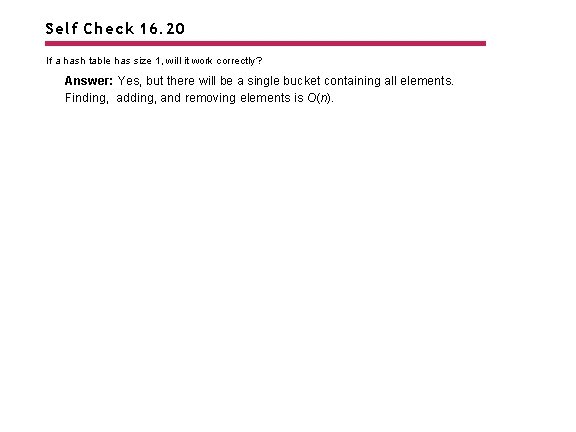Self Check 16. 20 If a hash table has size 1, will it work correctly? Answer: Yes, but there will be a single bucket containing all elements. Finding, adding, and removing elements is O(n).Self Check 16. 21 Suppose you have two hash tables, each with n elements. To find the elements that are in both tables, you iterate over the first table, and for each element, check whether it is contained in the second table. What is the big-Oh efficiency of this algorithm? Answer: The iteration takes O(n) steps. Each step makes an O(1) containment check. Therefore, the total cost is O(n).Self Check 16. 22 In which order does the iterator visit the elements of the hash table? Answer: Elements are visited by increasing (compressed) hash code. This ordering will appear random to users of the hash table.Self Check 16. 23 What does the has. Nextmethod of the Hash. Set. Iteratordo when it has reached the end of a bucket? Answer: It locates the next bucket in the bucket array and points to its first element.Self Check 16. 24 Why doesn’t the iterator have an addmethod? Answer: In a set, it doesn’t make sense to add an element at a specific position.